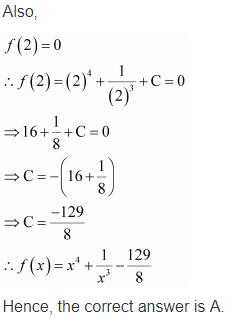# NCERT Solutions for Class 12 Maths Chapter 7 Integrals Ex 7.1

ncert textbook

## NCERT Solutions for Class 12 Maths Chapter 7 Integrals

The topics and sub-topics included in the Integrals chapter are the following:

 Section Name Topic Name 7 Integrals 7.1 Introduction 7.2 Integration as an Inverse Process of Differentiation 7.3 Methods of Integration 7.4 Integrals of some Particular Functions 7.5 Integration by Partial Fractions 7.6 Integration by Parts 7.7 Definite Integral 7.8 Fundamental Theorem of Calculus 7.9 Evaluation of Definite Integrals by Substitution 7.10 Some Properties of Definite Integrals

NCERT Solutions for Class 12 Maths Chapter 7  Integrals: Engineering aspirants and students appearing for CBSE Class 12 board exams must take the NCERT Mathematics textbooks seriously and finish them from top to bottom. They must also go through the NCERT solutions for Class 12 Maths and Class 11 Maths to have a better understanding of the various concepts. In this article, we will provide you with NCERT Solutions for Class 12 Maths Chapter 7 – Integrals which have been designed by the best teachers in India.

### Class 12 Maths Chapter 7 NCERT Solutions Integrals

NCERT Solutions for Class 12 Maths Chapter 7 – Integrals contains step-by-step and detailed solutions for every question.

1. Introduction
2. Integration as an Inverse Process of Differentiation
3. Geometrical interpretation of indefinite integral
4. Some properties of indefinite integral
5. Comparison between differentiation and integration
6. Methods of Integration
7. Integration by substitution
8. Integration using trigonometric identities
9. Integrals of Some Particular Functions
10. Integration by Partial Fractions
11. Integration by Parts
12. Integral of the type
13. Integrals of some more types
14. Definite Integral
15. Definite integral as the limit of a sum
16. Fundamental Theorem of Calculus
17. Area function
18. First fundamental theorem of integral calculus
19. Second fundamental theorem of integral calculus
20. Evaluation of Definite Integrals by Substitution
21. Some Properties of Definite Integrals

Question 1.
sin 2x
Solution: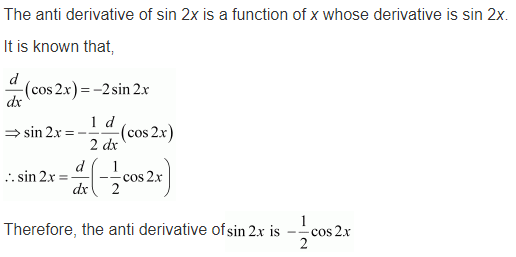Question 2.
cos 3x
Solution: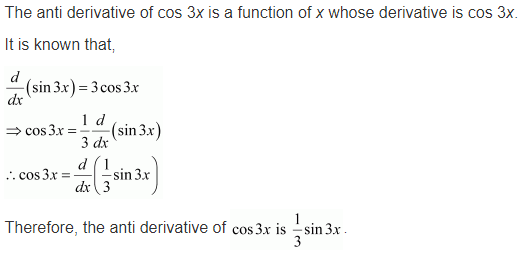Question 3.${ e }^{ 2x }$
Solution:Question 4.
(ax + c)²
Solution:Question 5.${ sin\quad 2x-4e }^{ 3x }$
Solution: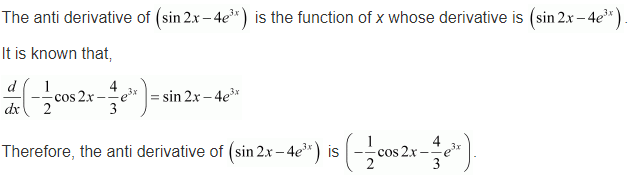Find the following integrals in Exercises 6 to 20 :

Question 6.$\int { \left( { 4e }^{ 3x }+1 \right) dx }$
Solution: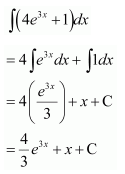Question 7.$\int { { x }^{ 2 }\left( 1-\frac { 1 }{ { x }^{ 2 } } \right) dx }$
Solution: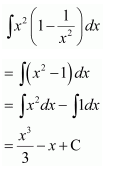Question 8.$\int { { (ax }^{ 2 }+bx+c)dx }$
Solution: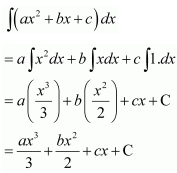Question 9.$\int { \left( { 2x }^{ 2 }+{ e }^{ x } \right) dx }$
Solution:Question 10.$\int { { \left[ \sqrt { x } -\frac { 1 }{ \sqrt { x } } \right] }^{ 2 }dx }$
Solution:Question 11.$\int { \frac { { x }^{ 3 }+{ 5x }^{ 2 }-4 }{ { x }^{ 2 } } dx }$
Solution: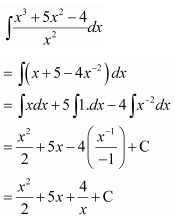Question 12.$\int { \frac { { x }^{ 3 }+3x+4 }{ \sqrt { x } } dx }$
Solution: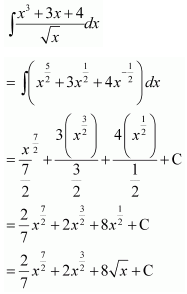Question 13.$\int { \frac { { x }^{ 3 }-{ x }^{ 2 }+x-1 }{ x-1 } dx }$
Solution:Question 14.$\int { \left( 1-x \right) \sqrt { x } dx }$
Solution:Question 15.$\int { \sqrt { x } \left( { 3x }^{ 2 }+2x+3 \right) dx }$
Solution: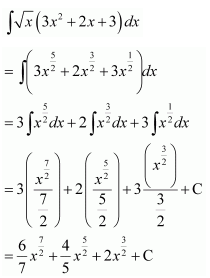Question 16.$\int { (2x-3cosx+{ e }^{ x })dx }$
Solution:Question 17.$\int { \left( { 2x }^{ 2 }-3sinx+5\sqrt { x } \right) dx }$
Solution:Question 18.$\int { secx(secx+tanx)dx }$
Solution: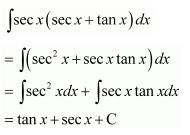Question 19.$\int { \frac { { sec }^{ 2 }x }{ { cosec }^{ 2 }x } dx }$
Solution: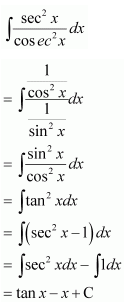Question 20.$\int { \frac { 2-3sinx }{ { cos }^{ 2 }x } dx }$
Solution: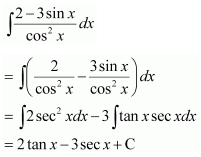Choose the correct answer in Exercises 21 and 22.
Question 21.
The antiderivative$\left( \sqrt { x } +\frac { 1 }{ \sqrt { x } } \right)$equals
(a)$\frac { 1 }{ 3 } { x }^{ \frac { 1 }{ 3 } }+{ 2x }^{ \frac { 1 }{ 2 } }+c$
(b)$\frac { 2 }{ 3 } { x }^{ \frac { 2 }{ 3 } }+{ \frac { 1 }{ 2 } x }^{ 2 }+c$
(c)$\frac { 2 }{ 3 } { x }^{ \frac { 3 }{ 2 } }+{ 2x }^{ \frac { 1 }{ 2 } }+c$
(d)$\frac { 3 }{ 2 } { x }^{ \frac { 3 }{ 2 } }+\frac { 1 }{ 2 } { x }^{ \frac { 1 }{ 2 } }+c$
Solution:Question 22.
If$\frac { d }{ dx } f(x)={ 4x }^{ 3 }-\frac { 3 }{ { x }^{ 4 } }$such that f(2)=0 then f(x) is
(a)${ x }^{ 4 }+\frac { 1 }{ { x }^{ 3 } } -\frac { 129 }{ 8 }$
(b)${ x }^{ 3 }+\frac { 1 }{ { x }^{ 4 } } +\frac { 129 }{ 8 }$
(c)${ x }^{ 4 }+\frac { 1 }{ { x }^{ 3 } } +\frac { 129 }{ 8 }$
(d)${ x }^{ 3 }+\frac { 1 }{ { x }^{ 4 } } -\frac { 129 }{ 8 }$
Solution: